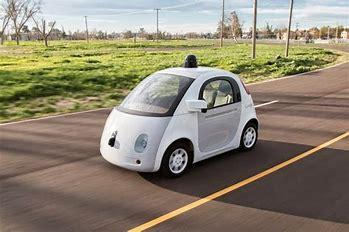• 框架

Tensorforce是架构在TensorFlow上的强化学习API，最早在17年就开源了，并发布了博客介绍其背后的设计思想及基本应用，国内机器之心将博客翻译成了中文：基于TensorFlow打造强化学习API：TensorForce是怎样炼成的？推荐阅读一下该文章，但文中的代码包括官方文档已经过时，相应的接口发生了变化，常见的接口会在后文介绍。

Environment <-> Runner <-> Agent <-> Model

Agent在训练前可以加载model，训练后保存model，运行时接收state，(预处理后)作为model的输入，返回action。模型会根据算法及配置自动更新。Runner将Agent和Environment之间的交互抽象出来作为函数。Environment根据应用场景的不同需要自己写接口，后文会提供一个机器人导航环境的接口案例。如果是学习或者算法测试，可以使用现成的基准环境，TensorForce提供了OpenAI Gym、OpenAI Universe和DeepMind Lab的接口。

• 第一行代码

from tensorforce.agents import PPOAgent
from tensorforce.execution import Runner
from tensorforce.contrib.openai_gym import OpenAIGym
# Create an OpenAIgym environment.
environment = OpenAIGym('CartPole-v0', visualize=True)
network_spec = [
dict(type='dense', size=32, activation='relu'),
dict(type='dense', size=32, activation='relu')
]
agent = PPOAgent(
states=environment.states,
actions=environment.actions,
network=network_spec,
step_optimizer=dict(
learning_rate=1e-3
),
summarizer=dict(directory='./record/', labels=["losses", "entropy"], seconds=600),
)
# Create the runner
runner = Runner(agent=agent, environment=environment)
# Start learning
runner.run(episodes=600, max_episode_timesteps=200)
runner.close()

# Query the agent for its action decision
action = agent.act(state)
# Execute the decision and retrieve the current information
observation, terminal, reward = GazeboMaze.execute(action)
# Pass feedback about performance (and termination) to the agent
agent.observe(terminal=terminal, reward=reward)

• 视频游戏

TensorForce提供了丰富的observation预处理功能，视频游戏是DRL最先取得突破的方向，以Flappy Bird为例，需要进行四个步骤的预处理：1. 转化为灰度图，去除不必要的颜色信息；2. 缩小输入的游戏截屏，resize为80*80；3. 堆叠连续的四帧，推导出运行信息；4. 归一化处理，以便于训练。

states = dict(shape=(3264, 18723, 3), type='float')
states_preprocessing_spec = [
dict(
type='image_resize',
width=80,
height=80),
dict(
type='grayscale' ),
dict(
type='normalize')
dict(
type='sequence',
length=4)
]

agent = DQNAgent(
states=states,
actions=actions,
network=network_spec,
states_preprocessing=states_preprocessing_spec)
• 环境搭建

• 复杂网络

DRL网络和算法是智能体最重要的两部分，一个确定模型结构、一个决定模型更新。上面只介绍了简单的模型，对于有些复杂网络需要层叠的方式来定义，如下如

states = dict(
image=dict(shape=(48, 64, 3), type='float'), # Observation
previous_act=dict(shape=(2,), type='float'), # velocity
relative_pos=dict(shape=(2,), type='float')  # target
)

dict(linear_vel=dict(shape=(), type='float', min_value=0.0, max_value=1.0),
angular_vel=dict(shape=(), type='float', min_value=-1.0, max_value=1.0))

network_spec = [
[
dict(type='input', names=['image']),
dict(type='conv2d', size=32, window=(8, 6), stride=4, activation='relu', padding='SAME'),
dict(type='conv2d', size=64, window=(4, 3), stride=2, activation='relu', padding='SAME'),
dict(type='conv2d', size=64, window=2, stride=2, activation='relu', padding='SAME'),
dict(type='flatten'),
dict(type='output', name='image_output')
],
[
dict(type='input', names=['image_output', 'previous_act', 'relative_pos'],
aggregation_type='concat'),
dict(type='dense', size=512, activation='relu'),
dict(type='dense', size=512, activation='relu'),
]
]

• 其它

1. agent会有actions_exploration参数来定义exploration，默认值为'none'，但这并不代表不探索，以PPO为例，模型在输出的时候不输出直接的确定性动作（只有DPG才会输出确定性动作），而是分布，输出在分布上采样输出，这可以看作是一种exploration。

2. 网络的输出层是根据action自动添加的，在network中定义输入层和隐藏层即可

3. 如果不再需要exploration而只是exploition，则运行:

agent.act(action, deterministic=True)

agent.act(action, independent=True)

4. PPO算法相比于DQN，不仅性能好，并且对超参数更鲁棒，建议优先选择PPO